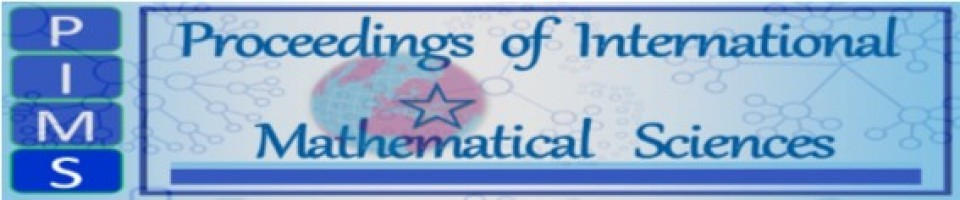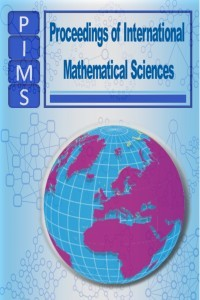##### Proceedings of International Mathematical SciencesMakale Gönder Hakemlik İsteği Gönder Amaç ve Kapsam Yazım Kuralları Etik İlkeler ve Yayın Politikası Son Sayı Editör Kurulu İletişim Arşiv
ISSN 2717-6355 | Yayın Aralığı Yılda 2 Sayı | Başlangıç: 2019 | Yayıncı İbrahim ÇANAK | https://dergipark.org.tr/tr/pub/pims

Proceedings of International Mathematical Sciences (PIMS) accepts research papers in all areas of Mathematical Sciences and its numerous applications.

Topics covered by the journal include: Topology, Complex Analysis, Functional Analysis, Sequences, series, summability, Fixed Point Theory, Numerical Functional Analysis, Computer Science and Technology, Mathematical Methods in Physics, Recent themes on Controllability and Stability of PDE's, Applied Statistics, Geometry, Mathematical Education, Algebra and Number Theory, Numerical analysis, Statistics, Differential equations, Difference equations, Partial differential equations, Optimization, Fourier analysis, Abstract Harmonic Analysis, Numerical Methods in Fourier Analysis, Numerical Functional Analysis, Operator Theory, Miscellaneous applications of functional analysis, Nonlinear functional analysis, Stochastic analysis, Multivariate analysis and all the other fields of their applications.

## Proceedings of International Mathematical Sciences

ISSN 2717-6355 | Yayın Aralığı Yılda 2 Sayı | Başlangıç: 2019 | Yayıncı İbrahim ÇANAK | https://dergipark.org.tr/tr/pub/pimsProceedings of International Mathematical Sciences (PIMS) accepts research papers in all areas of Mathematical Sciences and its numerous applications.

Topics covered by the journal include: Topology, Complex Analysis, Functional Analysis, Sequences, series, summability, Fixed Point Theory, Numerical Functional Analysis, Computer Science and Technology, Mathematical Methods in Physics, Recent themes on Controllability and Stability of PDE's, Applied Statistics, Geometry, Mathematical Education, Algebra and Number Theory, Numerical analysis, Statistics, Differential equations, Difference equations, Partial differential equations, Optimization, Fourier analysis, Abstract Harmonic Analysis, Numerical Methods in Fourier Analysis, Numerical Functional Analysis, Operator Theory, Miscellaneous applications of functional analysis, Nonlinear functional analysis, Stochastic analysis, Multivariate analysis and all the other fields of their applications.